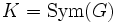# Every group is a subgroup of a complete group

This article gives the statement, and possibly proof, of an embeddability theorem: a result that states that any group of a certain kind can be embedded in a group of a more restricted kind.
View a complete list of embeddability theorems

## Statement

Let$G$ be a group. Then, there exists a complete group$H$ such that$G \le H$.

## Definitions used

Further information: Complete group

A group is termed complete if it satisfies the following two conditions:

## Facts used

1. Cayley's theorem: Every group is a subgroup of a symmetric group -- in fact, of the symmetric group on its underlying set.
2. Symmetric groups on finite sets are complete: The symmetric group on a finite set of size$n$ is a complete group if$n \ne 2, 6$.
3. Symmetric groups on infinite sets are complete

## Proof

Given: A group$G$.

To prove:$G$ is a subgroup of a complete group.

Proof: Let$K = \operatorname{Sym}(G)$. By Cayley's theorem (fact (1)),$G$ is a subgroup of$K$. We make two cases:

• The order of$G$ is not equal to$2$ or$6$: In this case facts (2) and (3) tell us that$K$ is a complete group, and we are done.
• The order of$G$ is equal to$2$ or$6$: In this case, let$H$ be the symmetric group on the set$G \sqcup \{ x_0 \}$, so$G \le K \le H$. Further,$H$ is the symmetric group on a set of size$3$ or$7$, which is complete, so$G$ is a subgroup of a complete group.